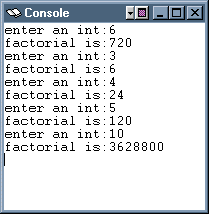An optimizing compiler might output assembly code that eliminates the intermediate variable `a`, as if the programmer had written:

``` main()
{
print(  mysub( 6 ) );
}
```

# Example Program: Factorial( N )

The next example program prompts the user for an integer, reads in the integer, and prints the factorial. The SPIM console window shows the output of several runs of the program. The pseudo-code for the program is:

```#  main()
#  {
#    int a, b;  // a: 0(\$fp),  b: 4(\$fp)
#    write("enter an int:")
#    b = fact( a );
#    write("factorial is:")
#    print( b );
#  }

#  int fact( int n )
#  {
#    if ( n <= 1 )
#      return 1;
#    else
#      return n*fact(n-1);
#  }
```

The math definition of factorial is:

```fact( n ) = 1, if n <= 1

= n * fact( n-1 ), otherwise
```

### QUESTION 17:

You have very likely seen the factorial function before (and are very likely sick of it!) But just in case:

What is the factorial of 5?